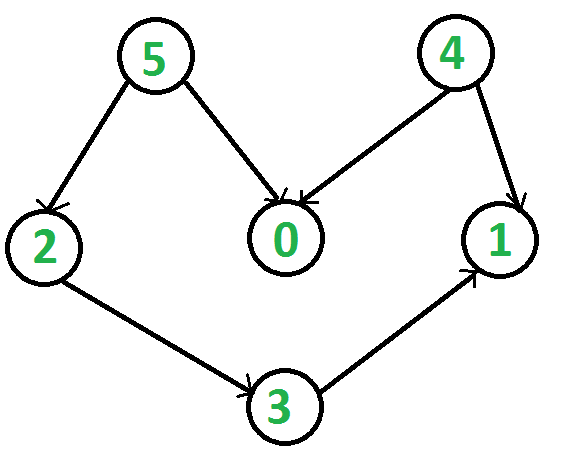# Print all possible paths in a DAG from vertex whose indegree is 0

Given a Directed Acyclic Graph (DAG), having N vertices and M edges, The task is to print all path starting from vertex whose in-degree is zero.

Indegree of a vertex is the total number of incoming edges to a vertex.

Example:

Input: N = 6, edges[] = {{5, 0}, {5, 2}, {4, 0}, {4, 1}, {2, 3}, {3, 1}}
Output: All possible paths:
4 0
4 1
5 0
5 2 3 1
Explaination:
The given graph can be represented as:There are two vertices whose indegree are zero i.e vertex 5 and 4, after exploring these vertices we got the fallowing path:
4 -> 0
4 -> 1
5 -> 0
5 -> 2 -> 3 -> 1
Input: N = 6, edges[] = {{0, 5}}
Output: All possible paths:
0 5
Explaination:
There will be only one possible path in the graph.

Approach:

The above problem can be solve by exploring all indegree 0 vertices and printing all vertices in path until a vertex having outdegree 0 is encountered. Follow these steps to print all possible paths:

1. Create a boolean array indeg0 which store a true value for all those vertex whose indegree is zero.
2. Apply DFS on all those vertex whose indegree is 0.
3. Print all path starting from a vertex whose indegree is 0 to a vertex whose outdegrees are zero.

Illustration:
For the above graph:
indeg0[] = {False, False, False, False, True, True}
Since indeg = True, so appling DFS on vertex 4 and printing all path terminating to 0 outdegree vertex are as follow:
4 -> 0
4 -> 1

Also indeg = True, so appling DFS on vertex 5 and printing all path terminating to 0 outdegree vertex are as follow:
5 -> 0
5 -> 2 -> 3 -> 1

Here is the implementation of the above approach:

## Python3

 `# Python program to print all ` `# possible paths in a DAG ` ` `  `# Recursive function to print all paths ` `def` `dfs(s): ` `    ``# Append the node in path ` `    ``# and set visited ` `    ``path.append(s) ` `    ``visited[s] ``=` `True` ` `  `    ``# Path started with a node ` `    ``# having in-degree 0 and ` `    ``# current node has out-degree 0, ` `    ``# print current path ` `    ``if` `outdeg0[s] ``and` `indeg0[path[``0``]]: ` `        ``print``(``*``path) ` ` `  `    ``# Recursive call to print all paths ` `    ``for` `node ``in` `adj[s]: ` `        ``if` `not` `visited[node]: ` `            ``dfs(node) ` ` `  `    ``# Remove node from path ` `    ``# and set unvisited ` `    ``path.pop() ` `    ``visited[s] ``=` `False` ` `  ` `  `def` `print_all_paths(n): ` `    ``for` `i ``in` `range``(n): ` ` `  `        ``# for each node with in-degree 0 ` `        ``# print all possible paths ` `        ``if` `indeg0[i] ``and` `adj[i]: ` `            ``path ``=` `[] ` `            ``visited ``=` `[``False``] ``*` `(n ``+` `1``) ` `            ``dfs(i) ` ` `  ` `  `# Driver code ` `from` `collections ``import` `defaultdict ` ` `  `n ``=` `6` `# set all nodes unvisited ` `visited ``=` `[``False``] ``*` `(n ``+` `1``) ` `path ``=` `[] ` ` `  `# edges = (a, b): a -> b ` `edges ``=` `[(``5``, ``0``), (``5``, ``2``), (``2``, ``3``), ` `         ``(``4``, ``0``), (``4``, ``1``), (``3``, ``1``)] ` ` `  `# adjacency list for nodes ` `adj ``=` `defaultdict(``list``) ` ` `  `# indeg0 and outdeg0 arrays ` `indeg0 ``=` `[``True``]``*``n ` `outdeg0 ``=` `[``True``]``*``n ` ` `  `for` `edge ``in` `edges: ` `    ``u, v ``=` `edge[``0``], edge[``1``] ` `    ``# u -> v ` `    ``adj[u].append(v) ` ` `  `    ``# set indeg0[v] <- false ` `    ``indeg0[v] ``=` `False` ` `  `    ``# set outdeg0[u] <- false ` `    ``outdeg0[u] ``=` `False` ` `  `print``(``'All possible paths:'``) ` `print_all_paths(n) `

Output:

```All possible paths:
4 0
4 1
5 0
5 2 3 1
```

Time Complexity: O (N + E)2
Auxiliary Space: O (N)

Attention reader! Don’t stop learning now. Get hold of all the important DSA concepts with the DSA Self Paced Course at a student-friendly price and become industry ready.

My Personal Notes arrow_drop_upCheck out this Author's contributed articles.

If you like GeeksforGeeks and would like to contribute, you can also write an article using contribute.geeksforgeeks.org or mail your article to contribute@geeksforgeeks.org. See your article appearing on the GeeksforGeeks main page and help other Geeks.

Please Improve this article if you find anything incorrect by clicking on the "Improve Article" button below.